Subsections

# Entropy, Energy and Free Energy in Canonical Ensemble

## Averaging Entropy

We know how to calculate mean energy. How can we calculate mean entropy ?

Consider a macroscopic state with energy E in canonical ensemble:SA = SM - SB

Probability of a microscopic state in canonical ensemble: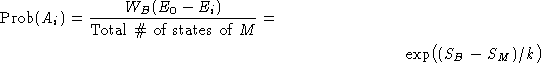We obtained: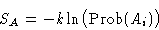Entropy of a macroscopic state with energy E in a canonical ensemble equals minus k log of the distribution function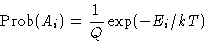Average entropy: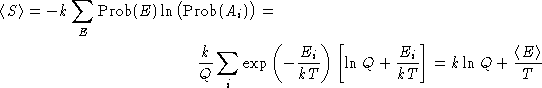Since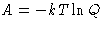, we obtained:

A = E - TS

We know that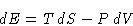and since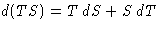,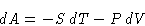## Aside: Legendre Transformations

Suppose we have a function f(x). New variables: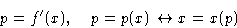Consider the function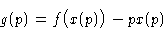We see that: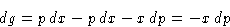or

g'(p) = -x

The function g is Legendre transformation of the function f.

Example: Hamilton & Lagrange Mechanics.

Free energy is Legendre transformation of energy (or entropy). In particular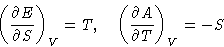It means that A=A(T,V)Next: Grand Canonical Ensemble Up: Ensembles and Thermodynamic Potentials Previous: Aside: Quiz Solutions

© 1997 Boris Veytsman and Michael Kotelyanskii
Tue Sep 9 22:39:08 EDT 1997